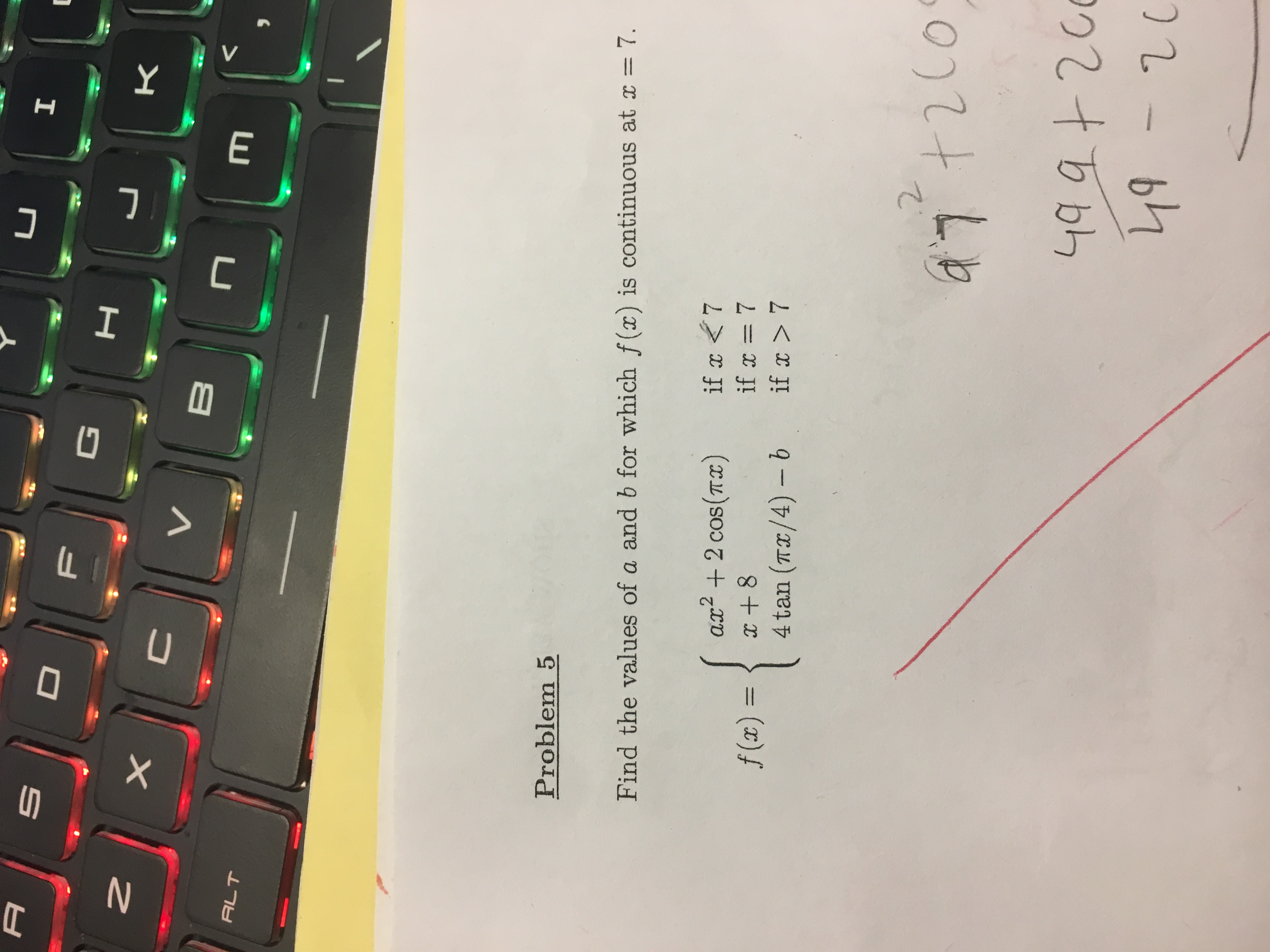# IADFкXVBALTProblem 5Find the values of a and b for which f(x) is continuous at x 7if x7ax2 cos(Tx)f (x) =14 tan (TI/4) - b2Co+20049 9420049-20VE-7U

Question
3 viewshelp_outlineImage TranscriptioncloseI A D F к X V B ALT Problem 5 Find the values of a and b for which f(x) is continuous at x 7 if x<7 if x= 7 if x >7 ax2 cos(Tx) f (x) = 1 4 tan (TI/4) - b 2 Co +200 49 94200 49-20 V E -7 U fullscreen
check_circle

Step 1

Consider the given function

Step 2

Now when x=7 then

Step 3

Then

For the function f(x) to be continuous must be 15...

### Want to see the full answer?

See Solution

#### Want to see this answer and more?

Solutions are written by subject experts who are available 24/7. Questions are typically answered within 1 hour.*

See Solution
*Response times may vary by subject and question.
Tagged in

### Continuity